# Integers Class 6 Maths Notes

In this page we have Notes for Integers |Class 6 Maths . Hope you like them and do not forget to like , social share and comment at the end of the page.

## What are Natural numbers?

• Counting numbers 1, 2, 3, 4, ...... etc. are called Natural numbers.
• The smallest natural number is 1
• There is no largest natural number.

## What is Whole Numbers?

• When zero (0) is added to the collection of natural numbers, then the numbers 0, 1, 2, 3, 4, ...... etc are called whole numbers
• The smallest whole number is 0
• There is no largest whole number.

## What is Integers

• Integers are a set of numbers that consist of all natural numbers, their negatives, and the number zero
• -4, -3, -2, -1, 0, 1, 2, 3, ………. etc are all integers

### Positive Integers and Negative Integers

• Positive integers are all the integers greater than zero.
• Negative integers are all the integers less than zero.

### Zero

Zero is neither positive nor negative and it serves as a neutral element in many mathematical operations.

## Representation of Integers on a Number Line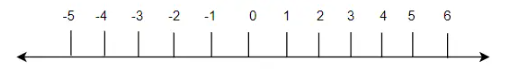A number line is a line which represent all the number.
How to draw the Number Line
• Draw a line and mark some points at equal distance on it as shown in the figure.
• Mark a point as zero on it.
• Points to the right of zero are positive integers and are marked + 1, + 2, + 3, etc. or simply 1, 2, 3 etc.
• Points to the left of zero are negative integers and are marked – 1, – 2, – 3 etc
• In order to locate negative integers, we move to the left
• In order to locate positive integers, we move to the Right

## Comparison or Ordering of Integers

• We can do comparison or ordering of Integers using Number line
• Integers on the right is greater than integers on the left
• The number increases as we move to the right and decreases as we move to the left
Example
0 > -3
6 > 2
-2 > -3

(1) When one positive and one negative integers are added, we subtract the smaller number from bigger number and put the sign of the bigger integer. The bigger integer is decided by ignoring the signs of the integers
For example, $(+10) + (-4) = + 6$ and $(-12) + (+ 1) = - 11$
(2) When two negative integers are added, we add both the number and put the negative sign on the number=
For example, $(-11) + (-5) = -16$ and $(-12) + (- 1) = -13$
(3)When a positive integer is added to an integer, the resulting integer becomes greater than the given integer.
(4)When a negative integer is added to an integer, the resulting integer becomes less than the given integer

## Addition of Two Integers on Number Line

1.We represent the First number on the Number
2.If a positive integer is being added, we move right to those many steps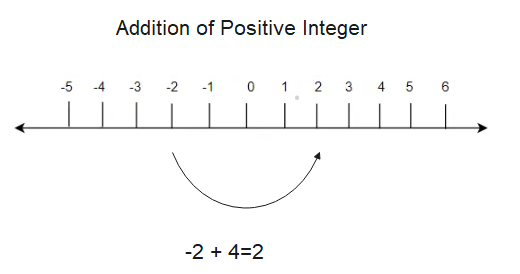3.If a Negative number is being added, we move left to those many steps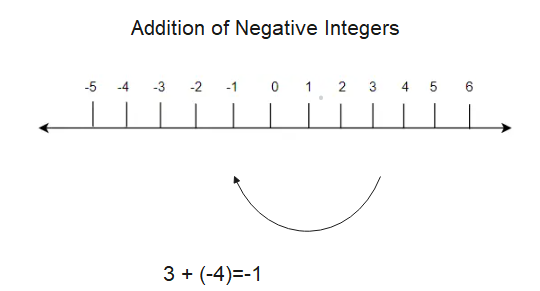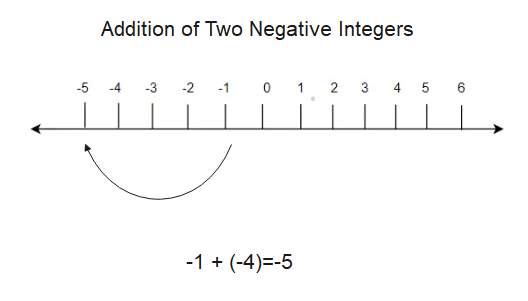Numbers such as 3 and – 3, 2 and – 2, when added to each other give the sum zero. They are called additive inverse of each other.

## Subtraction of Integers

To subtract an integer from another integer ,we need add the additive inverse of the integer that is being subtracted, to the other integer.
For example,
$(+10) - (-4) = (+10) + (4) =14$ and $(-12) - (+ 1) = (-12) + (-1)=-13$

## Subtraction of Two Integers on Number Line

1.We represent the First number on the Number
2.If a positive integer is being subtracted, we move left to those many steps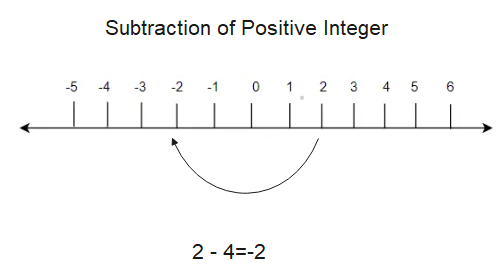3.If a Negative number is being subtracted, we move right to those many steps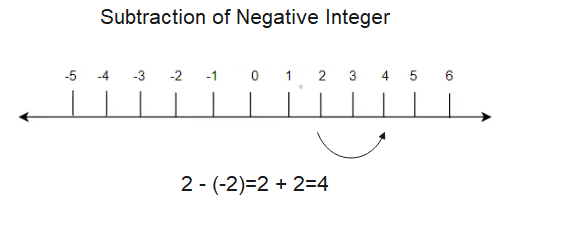### Practice Question

Question 1 What is $\frac {1}{2} + \frac {3}{4}$ ?
A)$\frac {5}{4}$
B)$\frac {1}{4}$
C)$1$
D)$\frac {4}{5}$
Question 2 Pinhole camera produces an ?
A)An erect and small image
B)an Inverted and small image
C)An inverted and enlarged image
D)None of the above